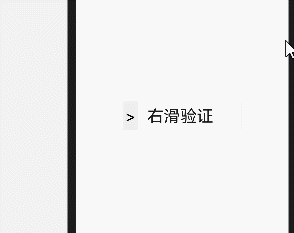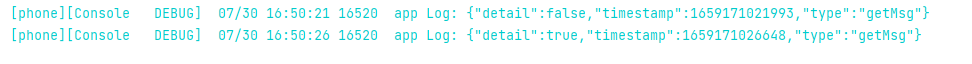# #夏日挑战赛# HarmonyOS 实现一个滑块验证 原创精华

lxj29

3收藏

### 效果展示• 第一次：没有滑倒最右侧，认证失败
• 第二次：滑动的速度太块，认证失败
• 第三次：滑动速度符合限制，认证成功

### 1.参数支持

width 滑块宽度 Number 300
height 滑块高度 Number 50
limit 滑块的速率限制 Number 4

### 2.事件支持

getMsg 验证通过或不通过事件### 原理分析

#### 1.滑动原理``````    touchstart (e) {
//设置X轴的始点
this.startPositionX = e.touches.localX;
this.startTime = 0
this.s = 0
this.num = 0
},
//滑块移动中执行的事件
touchmove (e) {
const offsetX = e.touches.localX - this.startPositionX
// 如果验证成功后仍允许滑动，则执行下面代码块（初始值默认为允许）
if (this.isSlide) {
//当x坐标大于最大可移动距离，那么当前x坐标即为最大可移动距离
if (offsetX >= this.max) {
this.x = this.max
} else {
// 将当前鼠标x坐标给滑块移动的距离
this.x = offsetX;
}
}
},
``````

#### 2.验证原理

``````    touchmove (e) {
const offsetX = e.touches.localX - this.startPositionX
const currTime = Date.now()
if (this.startTime !== 0) {
const duration = currTime - this.startTime
// 传入倒数第二个点和最后一个点，和持续时间，会返回加速度
const v = parseInt(offsetX / duration)
this.num++
this.s = this.s + v

}
this.startTime = currTime
// 如果验证成功后仍允许滑动，则执行下面代码块（初始值默认为允许）
if (this.isSlide) {
//当x坐标大于最大可移动距离，那么当前x坐标即为最大可移动距离
if (offsetX >= this.max) {
this.x = this.max
} else {
// 将当前鼠标x坐标给滑块移动的距离
this.x = offsetX;
}
}
},
//松开滑块执行的事件
touchend (e) {
//        计算平均速度
const avg = this.s / this.num
console.log(avg)
//自定义组件触发事件时提供的data对象
var data = {};
//如果触摸的X轴坐标大于等于限定的可移动范围，并且滑动的平均速度不能超过100，则验证成功
if (this.x == this.max && avg < this.limit) {
//设置验证成功提示语
this.status = '验证成功';
//设置data对象的返回值
data.msg = true;
//验证成功后，禁止滑块滑动
this.isSlide = false;
} else {
//元素X轴坐标归0
this.x = 0;
//清空验证成功提示语
this.status = '';
this.tip = '验证失败'
//设置data对象的返回值
data.msg = false;
}
this.\$emit('getMsg', data)
}
``````

### 完整代码

index.js

``````// @ts-nocheck
export default{
data:{
tip: '右滑验证',
x: 0,//X轴的初始值
startPositionX: 0,//触摸时X轴的值
max: 0,//滑块可移动的X轴范围
status: '',//验证完后的提示信息
isSlide: true,//是否允许验证成功后继续滑动
positionList: {}, // 存储坐标位置
startTime: 0,
num: 0, // 总次数
s: 0 // 速度之和
},
props: {
limit: {
type: Number,
default: 4
},
width: {
type: Number,
default: 400
},
height: {
type: Number,
default: 100
}
},
let ball = this.\$element("ball")
let box = this.\$refs.container
// 滑块可以移动的最大距离
this.max = box.getBoundingClientRect().width - ball.getBoundingClientRect().width
},
touchstart (e) {
//设置X轴的始点
this.startPositionX = e.touches.localX;
this.startTime = 0
this.s = 0
this.num = 0
},
//滑块移动中执行的事件
touchmove (e) {
const offsetX = e.touches.localX - this.startPositionX
const currTime = Date.now()
if (this.startTime !== 0) {
const duration = currTime - this.startTime
// 传入倒数第二个点和最后一个点，和持续时间，会返回加速度
const v = parseInt(offsetX / duration)
this.num++
this.s = this.s + v

}
this.startTime = currTime
// 如果验证成功后仍允许滑动，则执行下面代码块（初始值默认为允许）
if (this.isSlide) {
//当x坐标大于最大可移动距离，那么当前x坐标即为最大可移动距离
if (offsetX >= this.max) {
this.x = this.max
} else {
// 将当前鼠标x坐标给滑块移动的距离
this.x = offsetX;
}
}
},
//松开滑块执行的事件
touchend (e) {
//        计算平均速度
const avg = this.s / this.num
//自定义组件触发事件时提供的data对象
var msg = {};
//如果触摸的X轴坐标大于等于限定的可移动范围，并且滑动的平均速度不能超过100，则验证成功
if (this.x == this.max && avg < this.limit) {
//设置验证成功提示语
this.status = '验证成功';
//设置data对象的返回值
msg = true;
//验证成功后，禁止滑块滑动
this.isSlide = false;
} else {
//元素X轴坐标归0
this.x = 0;
//清空验证成功提示语
this.status = '';
this.tip = '验证失败'
//设置data对象的返回值
msg = false;
}
this.\$emit('getMsg', msg)
}
}
``````

### 总结📝

slider.zip 2.1K 20次下载

5

1条回复

/

2022-8-1 14:22:01帖子
视频
声望
粉丝
社区精华内容